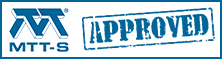# Voltage standing wave ratio (VSWR)

Click here to go to our discussion on mismatch loss (and other cool stuff!)

### VSWR

VSWR is defined as the ratio of the maximum voltage to the minimum voltage in standing wave pattern along the length of a transmission line structure. It varies from 1 to (plus) infinity and is always positive. Unless you have a piece of slotted line-test equipment this is a hard definition to use, especially since the concept of voltage in a microwave structure has many interpretations.

Sometimes VSWR is called SWR to avoid using the term voltage and to instead use the concept of power waves. This in turn leads to a mathematical definition of VSWR in terms of a reflection coefficient. A reflection coefficient is defined as the ratio of reflected wave to incident wave at a reference plane. This value varies from -1 (for a shorted load) to +1 (for an open load), and becomes 0 for matched impedance load. It is a complex number. This helps us because we can actually measure power.

The reflection coefficient, commonly denoted by the Greek letter gamma (Γ), can be calculated from the values of the complex load impedance and the transmission line characteristic impedance which in principle could also be a complex number.

Γ = (Zl - Z0)/(Zl + Z0)

The square of | Γ | is then the power of the reflected wave, the square hinting at a historical reference to voltage waves.

Now we can define VSWR (SWR) as a scalar value:

VSWR= (1 + | Γ |)/(1 - | Γ |) or in terms of s-parameters: VSWR= (1 + | S11 |)/(1 - | S11 |)

This is fine but what has it to do with common usage in ads and specifications. Generally, VSWR is sometimes used as a stand-in for a figure of merit for impedance matching. Sometimes this simplification of a scalar quantity and it’s restricted definition can lead to confusion in the matter of a source to load match. Most of the time there is no problem but, technically, VSWR derives from the ratio using the load impedance and the characteristic impedance of the transmission line in which the standing waves reside and not specifically to a source to load match. I prefer to think of VSWR as a figure of merit and to use the reflection coefficient whenever I am trying to solve problems.

By the way, if you think you have never experienced a standing wave personally, it's very unlikely. Standing waves in a microwave oven are the reason that food is cooked unevenly (the turntable is a partial solution to that problem). The wavelength of the 2.45 GHz signal is about 12 centimeters, or about five inches. Nulls in the radiation (and heating) will be separated at a distance similar to wavelength.

In some old microwave text books the Greek lowercase letter sigma (σ) is used to denote VSWR. We don't usually use this at Microwaves101.

Here's an index to our material on VSWR:

Standing waves in nature

Voltage standing waves

Visualizing VSWR

Other ways to express VSWR

Calculating VSWR from an impedance mismatch

### Standing waves in nature

What's a standing wave? Luckily there are tons of examples in nature. Any stringed instrument such as a guitar or piano makes music using standing waves. But what about a traveling wave that reflects off of an object and creates a standing wave due to constructive interference? Let's go to the beach. Breakers roll in off the ocean, come up on the sand, and disappear; no standing wave occurs. What's happening? The beach is absorbing all (or at least most) of the energy, in effect it is "matched" to the wave front. Now let's go next door to marina where all of those expensive yachts are moored... chances are there are vertical concrete seawalls inside the marina to allow owners to bring their boats close enough so that only a small walkway is needed to get to them. Now notice the breakwater that extends around the marina, with only a narrow opening for boats to go in and out. That's there because the vertical walls in the marina offer near perfect reflection to moving waves (an "open circuit"). Without the breakwater wall (which absorbs energy) huge standing waves are possible due to constructive interference, and all those boats would bob up and down like crazy corks and eventually everything would get smashed to tiny bits.

If you live in Arizona, lakes that were created by flooding canyons can offer excellent standing waves to for you to jump in your annoying personal watercraft... BY FAR the roughest water can be found on Lake Powell. Lake Powell was made by flooding Glen Canyon, and a large amount of its shoreline consists of literally vertical cliffs. This lake gets way rougher than Lake Mead, for example, with similar wind speeds. Lake Mead has longer and wider channels than Lake Powell (which should allow larger waves to build up), but most of its shoreline is gently sloped. The vertical walls of Lake Powell act as "open circuits" to the water waves, whereas the sloped beaches at Lake Mead act like "loads".

Enough talk about beaches, water and boats, it's summer, and we've got to get back to work!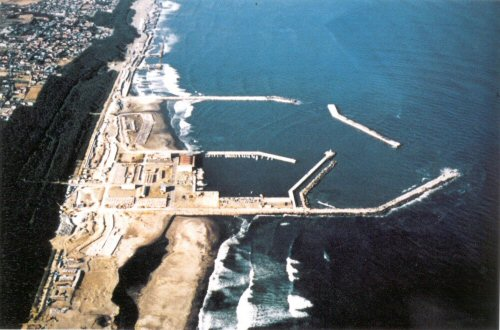Breakwater doing its job

## The best video ever made on wave behavior

This content was moved to a new page, for February 2021.  Click here.

We consider the stuff below obsolete but left it here because "it's not hurting nothin". Visit our page on visualizing VSWR for a better explanation!

We created an Excel file to show you how EM waves reflect and build up a standing wave. , You might find it useful for generating graphics for presentations. Just remember where you got it, it's in our download area.

In the next three plots, we illustrate how a standing wave arises at a change in transmission line impedance (a mismatch). In the first plot, pretend that there is a reflection coefficient of magnitude 0.3 at the X-value of 25. It this point, 70% of the wave continues on (blue trace) and 30% of the wave is reflected backwards (purple trace). The composite wave is the simple addition of the forward and backward waves at distance<25. The wave forms here are instantaneous, meaning that you are looking at a single moment frozen in time. In real life the waves are continuously moving.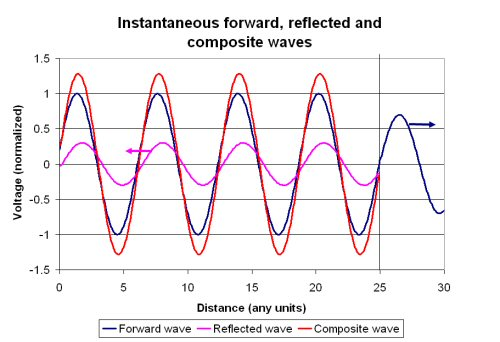Now let's look at 20 snapshots in time, equally spaced in time. At this point we will ignore the forward wave after the interface, and just look at the composite wave at distance<25. What's this, a pattern is emerging?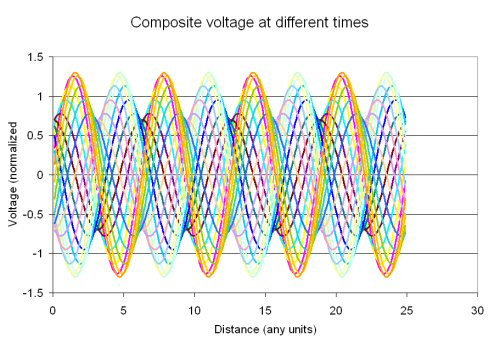Now using the "MAX" function of Excel, we can trap the maximum of all of the composites (just like a microwave detector would), and draw the standing wave: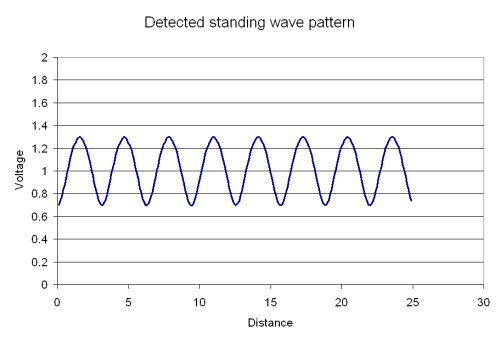From the Excel sheet, we get a peak of 1.299987 and a null of 0.697579. That's a standing wave ratio of 1.863569:1.

Let's check our math and recalculate the reflection coefficient from the Excel-generated VSWR:

ρ = magnitude of the reflection coefficient=(VSWR-1)/(VSWR+1)

ρ=(1.863569-1)/(1.863569+1)=0.301571

That's an error of less than 0.3 percent (the exact value of rho should be 0.3, remember?) Not bad considering we only "looked" at 10 snapshots in time.

For the record, let's look at the difference in wave patterns for a short circuit and an open circuit below (short circuit plot is first). Again, the mismatch is placed at X=25. Note that the minimum voltage of the standing wave in each case is zero, which means the standing wave ratio is infinite.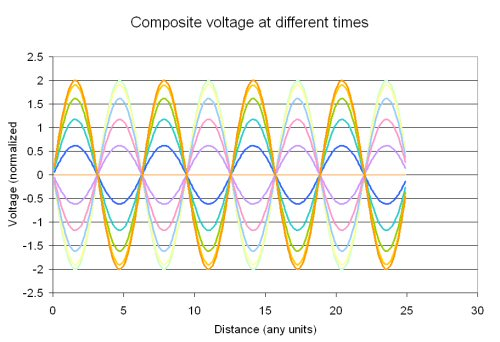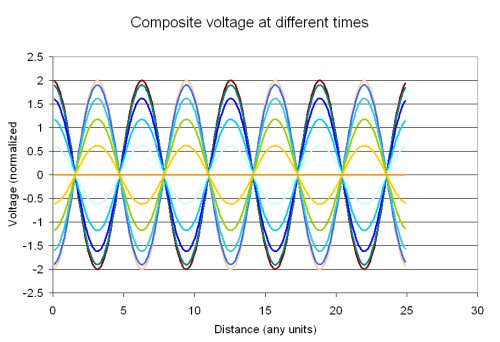Now you can see the difference, the waves all go to zero at a short circuit, and go to a maximum at an open circuit.

### Other ways to express impedance mismatches

The reflection coefficient is what you'd read from a Smith chart. A reflection coefficient with a magnitude of zero is a perfect match, a value of one is perfect reflection. The symbol for reflection coefficient is uppercase Greek letter gamma (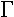). Note that the reflection coefficient is a complex value, so it includes an angle. Unlike VSWR, the reflection coefficient can distinguish between short and open circuits. A short circuit has a value of -1 (1 at an angle of 180 degrees), while an open circuit is one at an angle of 0 degrees. Quite often we refer to only the magnitude of the reflection coefficient. The symbol for this is the lower case Greek letter ρ.

The return loss of a load is merely the magnitude of the reflection coefficient expressed in decibels. The correct equation for return loss is:

Return loss = -20 x log [mag(Γ)]

Thus in its correct form, return loss will usually be a positive number. If it's not, you can usually blame measurement error. The exception to the rule is something with negative resistance, which implies that it is an active device (external DC power is converted to RF) and it is potentially unstable (it could oscillate). Not something you have to worry about if you are just looking at coax cables! However, many engineers often omit the minus sign and talk about "-9.5 dB return loss" for example. People that find it necessary to correct engineers who do this have underwear that is too tight.

Here are the equations that convert between VSWR, reflection coefficient (Γ) and return loss (RL) as well as mismatch loss (ML), which we will cover later). Note that |Γ| in these equations always stands for the magnitude of the complex reflection coefficient and is itself a scalar term in these equations: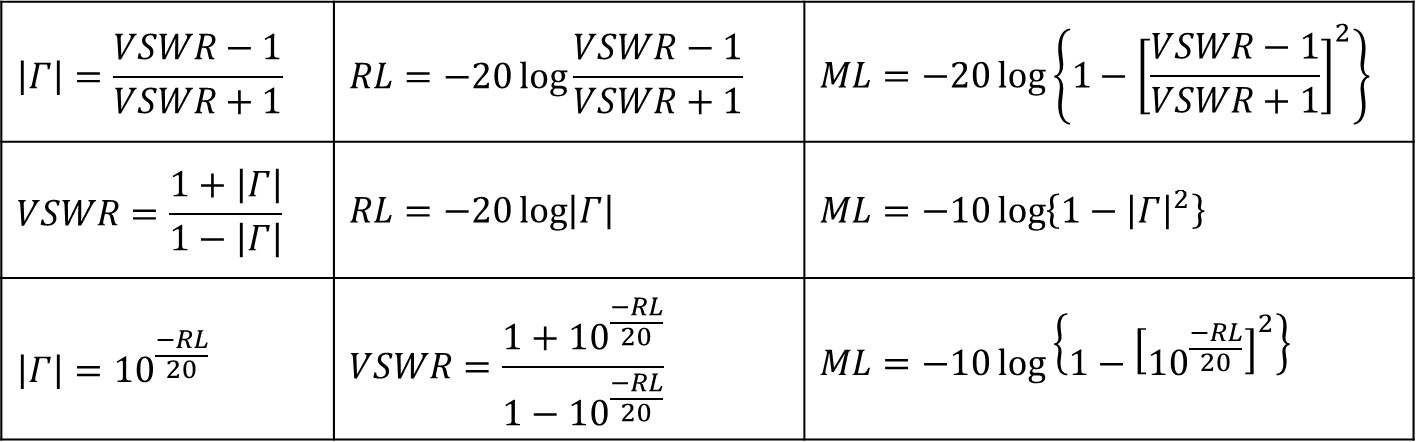Let's end our discussion with a table of reflection VSWR, refection coefficient and return loss values (and remember that our VSWR  calculator can provide any values you need). If you want to impress your friends, memorize as much of this table as you can. Yes, rounding off is permitted.

VSWR Reflection coefficient Return loss Notes
1:1 0.00 infinity a perfect match
1.1:1 0.05 26.44
1.2:1 0.09 20.83
1.3:1 0.13 17.69
1.4:1 0.17 15.56
1.5:1 0.20 13.98 A good rule of thumb: 1.5:1 = 14 dB
1.6:1 0.23 12.74
1.7:1 0.26 11.73
1.8:1 0.29 10.88
1.9:1 0.31 10.16 A good rule of thumb: 1.9:1 = 10 dB
2.0:1 0.33 9.54
3.0:1 0.50 6.02 A good rule of thumb: 3:1 = 6 dB
4.0:1 0.60 4.44
5.0:1 0.67 3.52
6.0:1 0.71 2.92
10:1 0.82 1.71
infinity:1 1.000 0.00 short or open circuit

### Calculating VSWR from impedance mismatches

The mismatch of a load ZL to a source Z0 results in a reflection coefficient of:

Γ=(ZL-Z0)/(ZL+Z0)

Note that the load can be a complex (real and imaginary) impedance. If you can't remember in which order the numerator is subtracted (did we just say "ZL-Z0" or Z0-ZL"?), you can always figure it out by remembering that a short circuit (ZL=0) is on the left side of the Smith chart (angle = -180 degrees) which means Γ=-1 in this case, which means that the minus sign belongs in front of Z0.

The magnitude of the reflection coefficient is given by:

ρ=mag(Γ)

For cases where ZL is a real number,

ρ=abs((ZL-Z0)/(ZL+Z0))

Note that "abs" means "absolute value" here. VSWR can be calculated from the magnitude of the reflection coefficient:

VSWR=(1+ρ)/(1-ρ)

For cases where ZL is real, with a little algebra you'll see there are two cases for VSWR, calculated from load impedance:

For ZL<Z0: VSWR=Z0/ZL

For ZL>Z0: VSWR=ZL/Z0

Just remember to divide the larger impedance by the smaller impedance, because VSWR is always greater than 1. Hey, this calculation is so easy you can do it in your head!!!

Let's look at the special case where you mix up 50 ohm parts into a 75 ohm system (or vice-versa). In either case, the resulting VSWR is 1.5:1. Yes, we did that without a calculator. While we're at it, the reflection coefficient is:

Γ=(75-50)/(75+50)=0.2

Author : Unknown Editor